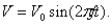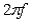# [Solved] The Output Voltage of an Ac Generator Is of the Form

Question 1
Multiple Choice

## The output voltage of an AC generator is of the formA typical frequency in household circuits is 60 Hz. Using radians for angular measure in this equation, what is the factorin radians per second for this frequency?

A) 2
B) 120
C) 377
D) 60

10+ million students use Quizplus to study and prepare for their homework, quizzes and exams through 20m+ questions in 300k quizzes.

### Physics & Astronomy

Explore our library and get Introductory Physics Homework Help with various study sets and a huge amount of quizzes and questions

120

Study sets

3.1K

Quizzes

233.2K

Questions

Upload material to get free accessInvite a friend and get free accessSubscribe and get an instant access